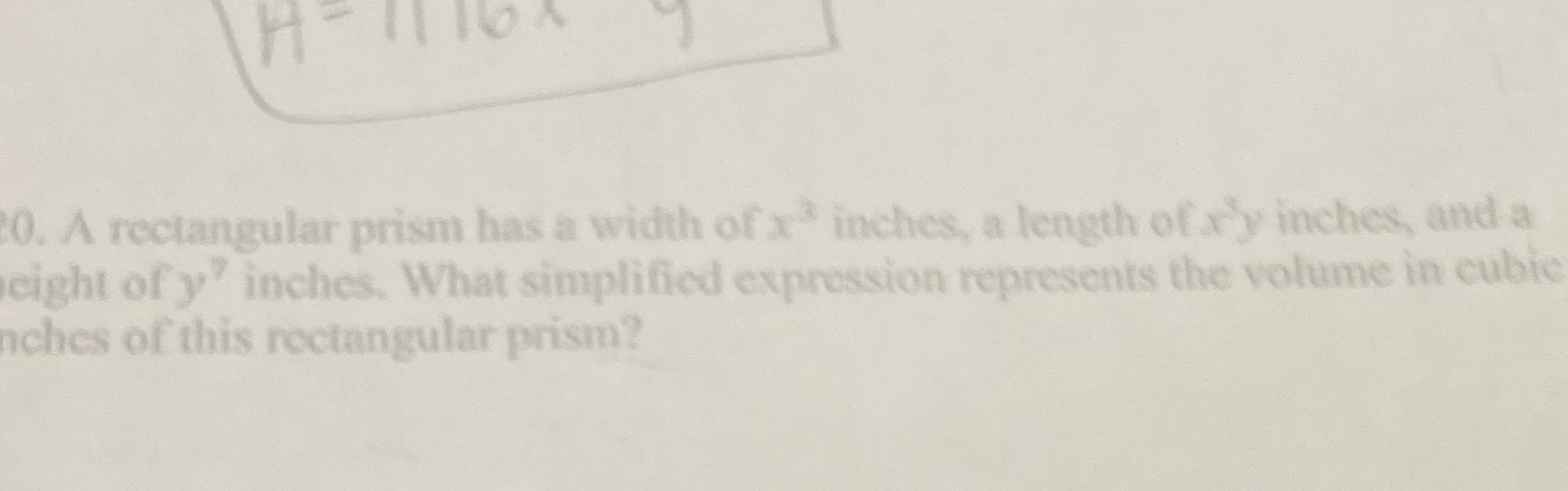### ¿Todavía tienes preguntas de matemáticas?

Pregunte a nuestros tutores expertos
Algebra
Pregunta0. A rectangular prism has a width of $$x ^ { 3 }$$ inches, a length of $$x ^ { 5 } y$$ inches, and a eight of $$y ^ { 7 }$$ inches. What simplified expression represents the volume in cubie nches of this roctangular prism?

volume = $$x^6y^8$$